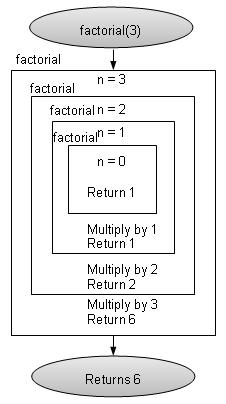# DSA using C - Recursion

#### Complete Python Prime Pack

9 Courses     2 eBooks

#### Artificial Intelligence & Machine Learning Prime Pack

6 Courses     1 eBooks

#### Java Prime Pack

9 Courses     2 eBooks

## Overview

Recursion refers to a technique in a programming language where a function calls itself. The function which calls itself is called a recursive method.

## Characteristics

A recursive function must posses the following two characteristics.

• Base Case(s)

• Set of rules which leads to base case after reducing the cases.

## Recursive Factorial

Factorial is one of the classical example of recursion. Factorial is a non-negative number satisfying following conditions.

• 0! = 1

• 1! = 1

• n! = n * n-1!

Factorial is represented by "!". Here Rule 1 and Rule 2 are base cases and Rule 3 are factorial rules.

As an example, 3! = 3 x 2 x 1 = 6

```int factorial(int n){
//base case
if(n == 0){
return 1;
} else {
return n * factorial(n-1);
}
}
```## Recursive Fibonacci Series

Fibonacci Series is another classical example of recursion. Fibonacci series a series of integers satisfying following conditions.

• F0 = 0

• F1 = 1

• Fn = Fn-1 + Fn-2

Fibonacci is represented by "F". Here Rule 1 and Rule 2 are base cases and Rule 3 are fibonnacci rules.

As an example, F5 = 0 1 1 2 3

## Example

```#include <stdio.h>

int factorial(int n){
//base case
if(n == 0){
return 1;
} else {
return n * factorial(n-1);
}
}
int fibbonacci(int n){
if(n ==0){
return 0;
}
else if(n==1){
return 1;
} else {
return (fibbonacci(n-1) + fibbonacci(n-2));
}
}
main(){
int n = 5;
int i;
printf("Factorial of %d: %d\n" , n , factorial(n));
printf("Fibbonacci of %d: " , n);
for(i=0;i<n;i++){
printf("%d ",fibbonacci(i));
}
}
```

## Output

If we compile and run the above program then it would produce following output −

```Factorial of 5: 120
Fibbonacci of 5: 0 1 1 2 3
```